# Modelling and Analysis of Solar and Wind System Adequacy Assessment and Cost Optimization

Modelling and Analysis of Solar and Wind System Adequacy Assessment and Cost Optimization
Vel Tech Rangarajan Dr. Sagunthala R&D Institute of Science and Technology, Department of Electrical and Electronics Engineering, Chennai 600062, India

Corresponding Author Email:
Page:
861-870
|
DOI:
https://doi.org/10.18280/mmep.080604
26 April 2021
|
Accepted:
19 August 2021
|
Published:
22 December 2021
| Citation

OPEN ACCESS

Abstract:

The output of wind and the solar system is not constant; it is difficult to access the adequacy of the system. The Generation model is developed for 240 MW of different generation units in the Roy Billinton Test System (RBTS). Then a multi-state wind and solar generation model is developed based on different solar radiation and wind speed to evaluate the probability of the states. In this work, the wind and solar systems are studies for separate locations with each consist of 8 MW, 18 MW, 28 MW, and 38 MW generation capacities. This wind and solar generation model are applied to Roy Billinton Test System (RBTS) to evaluate the reliability indices like Loss of Load Expectation (LOLE). Based on the development of the MATLAB program for determining reliability indices, the capacity outage probability is developed for multiple solar and wind states. Based on standard load forecasting and the Time Series Load forecasting technique, the reliability of the system is analyzed. The results reveal the variation of risk indices in the system when additional generators are incorporated into the RBTS generation system. The cost optimization for Solar and Wind system were conducted using HOMER software to obtain the levelized cost of the proposed system.

Keywords:

reliability, cost optimization, loss of load expectation, Roy Billinton test system, solar and wind

1. Introduction

1.1 Motivation

Green energies like solar and wind power generation give clean power generation to the environment. The output of renewable energies like Solar and Wind are not constant in nature due to changes in environmental conditions. Due to an outage in the renewable system, there will be a certain level of risk indices. The main motivation of this research is to analyze the risk indices of the renewable energy system incorporated with the RBTS test system and cost optimization. Solar radiation and wind speed are the main parameters for Forced outage Rate. By adding suitable generators, the system will meet the future load demand.

1.2 Literature survey

1.3 Contributions proposed in the paper

Since the wind and Solar system will not be available every time, the Forced Outage Rate (FOR) is an important factor to evaluate during system analysis. After knowing the FOR value of wind and the solar unit, the Capacity Outage Probability Table (COPT) has developed based on the proposed methodology of adequacy assessment. Then the 185 MW load duration Curve is drawn from the RBTS load data. Then the 240 MW RBTS test system is added to 8 MW, 18 MW, 28 MW, 38 MW solar/wind unit with different FOR values. Each unit consist of 2 MW then this is subdivided in to 0 MW, 0.25 MW, 0.5 MW, 0.75 MW, 1 MW, 1.25 MW, 1.5 MW, 1.75 MW, 2 MW as 9 states. Then the individual probability is evaluated for every case. The hourly load duration curve will start from 0 hrs to 8760 hrs. Then the reliability indices like Loss of Load Expectation (LOLE) of the system is calculated based on individual probability IPi and time Ti values. The cost optimization performed for Solar and Wind system.

2. Objective Function

The objective function is:

Minimize cost of the system $=\sum_{n}\left(C_{c a, a n n}+C_{r e, a n n}+C_{o m, a n n}\right)$

where, n is different components in the system like Solar Photovoltaic panels, Wind turbine, converter and battery, Cca,ann is annual Capital cost, Cre,ann is Annual replacement cost, Com,ann is Annual Operation and Maintenance cost.

Then the reliability of the system evaluated based on load forecasting technique. The amount of solar/wind power that can’t able to supply power to the load is known as Loss of Load Expectation. The Loss of Load Expectation can be calculated in Eq. (1).

$L O L E=\sum_{i=1}^{n}[I P i * t i] *(365 / 100)$    (1)

where,

IPi- Individual Probability of ith unit.

ti- Time in % for Load duration curve.

3. Proposed Methodology for Adequacy Assessment

$I P i=\frac{N !}{F O !(N-F O) !} W^{f} . Y^{N-F O}$    (2)

where, W = Unavailability, Y = Availability, FO = No of units on the forced outage, N = No of units.

## 1.png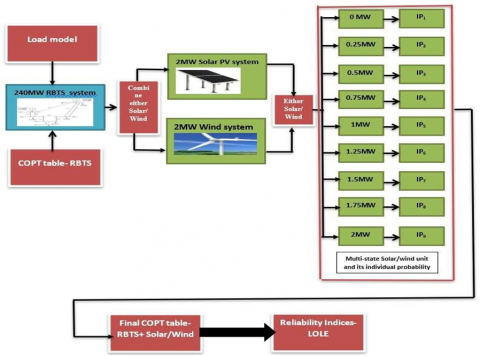Figure 1. Methodology for adequacy assessment

4.1 Generation model

In this section, the Roy Billinton Test System generation model and solar/wind generation model were discussed.

4.1.1 Roy Billinton Test System Generation model

The Roy Billinton Test System (RBTS) consist of two 5MW hydro generation units with FOR as 0.010 each, four 20MW hydro generation units with each 0.015 FOR each, one 20MW thermal generation units with 0.025 FOR, one 40MW hydro generation units with 0.020 FOR, two 40MW thermal generation unit with 0.030 FOR each, one 10MW thermal generation unit with FOR 0.020. There are a total of 11 generation units available in the RBTS test system. Based on the unavailability ‘W’ of each generation unit, the Individual Probability ‘IPi’ is evaluated. Then the Capacity Outage Probability Table developed for the RBTS generation system based on the methodology of adequacy assessment. Each state consists of 5MW, so a total of 49 states are evaluated in the COPT table to evaluate the expected loss of load. The Roy Billinton Test system representing the generation units is shown in Figure 2.

## 2.png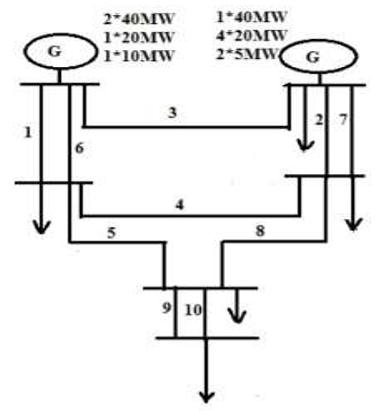Figure 2. RBTS generation system

4.1.2 Mathematical modeling of solar and wind generation

Solar radiation is an important parameter for evaluating solar output power. The Solar output power ‘PS’ is evaluated using the Eq. (3).

Solar output power PS = ηS * SN * SA * SR    (3)

where, SR= Average solar radiation, SA = Area of solar (m2), SN = Number of solar panels, ηS = Efficiency of the solar panel. The wind speed data is an important factor

The Wind output power ‘PWT’ is evaluated using the Eq. (4).

$P_{W T}=\left\{\begin{array}{lr}0 & 0 \leq w<w s c i \\ \operatorname{Pr} *(E+F * v+G * v * v) & w c i \leq w<w r \\ \operatorname{Pr} & w r \leq w<w c o \\ 0 & w \geq w c o\end{array}\right.$    (4)

where, w = wind speed (m/s), wci - Cut in wind speed (m/s), wco = Cut out wind speed (m/s), wr = Rated wind speed (m/s), Pr = Rated output power (MW), E,F,G = calculated from a cut in, cut out and rated wind speed.

Based on the output power of wind and solar PV systems, the Forced Outage Rate is calculated. The Forced outage Rate is 0.756 for solar and 0.749 for wind.

The Load is modeling based on standard load forecasting and time series load forecasting techniques. The procedure for this two-forecasting technique for assessing the adequacy is discussed in this section.

4.2.2 Procedure for time-series load forecasting technique

The Time series load forecasting is the technique used for forecasting the load in advance in which the set of load data is recorded for specified interval of time. By using this technique, the future load determined for evaluating future reliability indices. The Procedure for Time-series load forecasting technique as follow:

Step 1: Observe the load demand values at regular time.

Step 2: Determine the Latest load forecasting using in Eq. (5).

LFt= β(PLt-1- PFt-1)+ PFt-1    (5)

where,

LFt = Latest forecast,

PFt-1 = Past forecast,

β- Constant (0 ≤ β ≤1).

Step 3: The Error is defined as the difference between actual measurement and forecast for time ‘t’.

Evaluate Error during measurement using Eq. (6).

Error = Ωt- µt    (6)

Ωt-= Actual measurement for time period ‘t’.

µt-= Forecast for same time period ‘t’.

Step 4: The percentage error is the ratio of the difference between actual measurement ‘Ωt’ and forecast for the period ‘t’ ‘µt’ and forecast for the period ‘t’. The Error in % is represented in Eq. (7).

$\operatorname{Error} \%=\frac{\Omega t-\mu t}{\mu t}$    (7)

Step 5: The Average error is the average difference between actual measurement ‘Ωt’ and forecast for the period‘t’ ‘µt’. The average error is evaluated in Eq. (8).

Average error $=\frac{1}{\text { number of values }}+\sum_{t=0}^{n}(\Omega t-\mu t)$    (8)

Step 6: The Average square error is the average square of the difference between the actual measurements ‘Ωt’ and forecast for the period ‘t’ ‘µt’. The Average square error is evaluated in Eq. (9).

Average square error $=\frac{1}{\text { number of values }}+\sum_{t=0}^{n}(\Omega t-\mu t)^{2}$    (9)

Step 7: The Root average square error is the square root of average values in the square of the difference between the actual measurement ‘Ωt’ and forecast for the period ‘t’ ‘µt’. The Root Average square error is evaluated using (10).

Root Average square error $=\sqrt{\frac{1}{\text { number of values }}+\sum_{t=0}^{n}(\Omega t-\mu t)^{2}}$    (10)

Step 8: The Mean absolute percentage error is the average of percentage error and it is given in Eq. (11).

Mean Absolute % Error $=\frac{1}{\text { number of values }} \sum_{t=0}^{n} Error \%$     (11)

Step 9: The Standard error deviation is the average square of the difference between the average error and error. It is represented in Eq. (12).

Standard Error Deviation $=\sqrt{\frac{1}{\text { number of values }}+\sum_{t=0}^{n} \text { (Average error - error) }^{2}}$    (12)

5. Related Work

5.1 Error measurement analysis

The error is measured during the process of time series load forecasting. The load can be predicted in advance for several years. The absolute error measurement, Root Average Square Error, Maximum negative error, Maximum positive error is discussed in this section.

5.1.1 Measurement of error (absolute)

The error is measured during the processing of data. The value is measured and it is represented by ‘Xm’. The value which is already known was represented by ‘XK’. Then the difference between Xm and XK is known as Absolute error measurement. Based on the procedure of the time series load forecasting technique, the results are obtained as shown in Table 1. The maximum positive and negative error is 0.4 and -0.9. The mean absolute error is determined and it is 0.38. Between two variables when the result is not obtained between these two variables then in correlation measurement there is an error. The correlation obtained is in absolute error measurement is 0.999563. The coefficient of determination is forecasted from the independent variable. The coefficient of determination R2 = 99.8851%. Then the 99.8851% of the dependent variable variability is taken into account and 0.1149% of the residual variability is now undercounted.

Table 1. Measurement of error (absolute)

 Post Processed result Model fit Number of Observations 5 Maximum negative error -0.9 Maximum positive error 0.4 Mean absolute error 0.38 Root average square error 0.479583 Residual sum -0.5 The standard deviation of residuals 0.469042 Coefficient of determination (R2) 0.998851 Correlation 0.999563

5.1.2 Measurement of error (range %)

Table 2. Measurement of error (range %)

 Post Processed result Model fit Number of observations 5 Maximum negative error -2.25% Maximum positive error 1% Normalized average absolute error 0.95% Normalized root average square error 1.19896% Residual sum -1.25% The standard deviation of residuals 1.1726% Coefficient of determination (R2) 0.998851 Correlation 0.999563

The measurement of error is an important factor for analyzing better results. In the load time series load forecasting, the load was predicted in advance. The load is forecasted based on the previous values. There are different parameters analyzed based on the time series forecasting procedure as shown in Table 2. The maximum positive and negative error is determined as 1% and -2.25%. Then the average absolute error is 0.95%. The disparity between the data and an approximation mode is calculated in the residual sum. The residual sum is estimated as -1.25%. Then the standard deviation of the residuals is evaluated as 1.1726%. The latest forecast is evaluated for 9 years based on the observed value using the time-series load forecasting technique. The number of times an observation occurs in a given load forecast data is referred as number of occurrences. Since residual value determined as 0.25 with 5 number of occurrences, it is considered as final value. The measurement during time- series load forecasting, the residual value is obtained as shown in Figure 3.

## 3.pngFigure 3. Residual value in the measurement

5.1.3 Measurement of error (target %)

Based on the time series forecasting procedure, the error, different parameters are tabulated for measurement as shown in Table 3. There are five observations taken during load forecasting. The variables will be forecasted based on the observed value. There is an error in measurement due to the vast variation in the observed variables. The maximum positive and negative error during model fit is 0.196078% and -0.486486%. Then the root average square percentage error is 0.25%. The Standard deviation of residuals is 0.243475%.

Table 3. Measurement of error (target %)

 Post processed result Model fit Number of Observations 5 Maximum negative error -0.486486% Maximum positive error 0.196078% Average absolute % error 0.191184% Root average square % error 0.25% Residual sum -0.0488998% The standard deviation of residuals 0.243475% Coefficient of determination (R2) 0.998851 Correlation 0.999563

5.1.4 Evaluation of the latest forecast

The observed load demand for 5 years is taken as a variable to forecast the load. The minimum value of load demand is 185 MW and the maximum value of load demand is 225 MW. Based on this, the median, mean value, and standard deviation are evaluated and shown in Table 4.

 Variable System load Numeric values 5 Minimum value 185 MW Maximum value 225 MW Median 204 MW Mean value 204.5 Standard deviation 14.1483

There are different measurements P1 ≤ P ≤ Pn for different time intervals T1 ≤ T≤ Tn. The autocorrelation function is evaluated based on the lag‘f’ from the Eq. (13). Based on this Eq. (13). the autocorrelation curve is represented in Figure 4.

$r=\frac{\sum_{i=1}^{n-f}(\mathrm{Pi}-\mathrm{P})(\mathrm{Pi}+1-\mathrm{P})}{\sum_{i=1}^{n}(\mathrm{Pi}-\mathrm{P})^{2}}$    (13)

Let the time interval ‘T’ is assumed variable so that ‘T’ not evaluated in the above equation.

## 4.png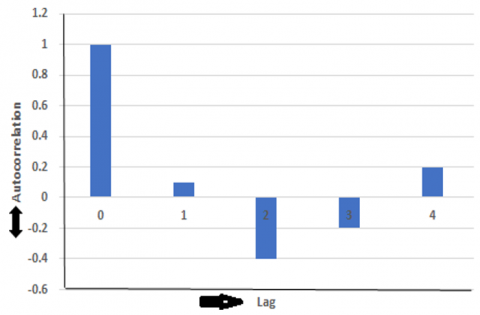Figure 4. Autocorrelation in time series load forecasting method

Table 5. Latest forecast result using Time series Load forecasting

 Year Actual Latest forecast Lower Upper Residuals 2020 185 184.1 183.14 185.05 -0.9 2021 194.3 194.25 193.29 195.20 4.76916 E-11 2022 204 204.4 203.44 205.35 0.4 2023 214.3 214.55 213.59 215.50 0.3 2024 225 224.7 223.74 225.65 -0.3 2025 - 234.85 233.89 235.80 - 2026 - 245 244.04 245.95 - 2027 - 255.15 254.19 256.10 - 2028 - 265.3 264.34 266.25 -

## 5.png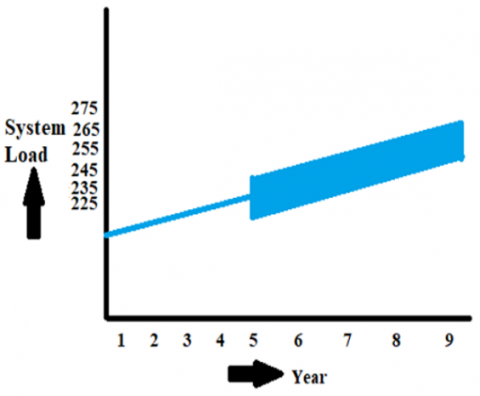Figure 5. Confidence band reliability analyze of solar and wind

The load demand for the first 5 years between 2020 and 2024 is given as input data. The observed actual data for the first 5 years are 185, 194.3, 204, 214.3, and 225. These values are represented in MW. Based on the observed values, the latest forecast is determined using the time series forecasting technique. There is some deviation in the perdition result compared to the observed value. For each year’s latest forecast, the upper and lower limit is determined. The corresponding residuals for the observed value are highlighted in the result. These latest forecasts are determined using the time series forecasting technique to evaluate the reliability of the system. These predicted values help to determine the reliability of the future system. The results of the latest forecast using the time series forecast technique are shown in Table 5. There is better accuracy in the result which is highlighted as the confidence band shown in Figure 5.

6. System Optimization Model for Solar and Wind System

The average solar radiation and wind speed was downloaded from the NASA data available in HOMER software for the particular location. The HOMER software gives the optimal solution and gives the least net present cost of the system. The optimization of cost obtained based on the objective function.

The Annualized cost is the total cost of the system, which includes capital cost, replacement cost and operating cost. This annualized cost can be calculated from Eq. (14).

Cann=QC*[(Cca,ann+Cre,ann)*FCR (IR,YN)]+Com,ann    (14)

The Capital Recovery factor FCR is represented in Eq. (15).

FCR(IR,YN)=[IR(1+IR)Yn]/[(1+IR)Yn-1]    (15)

where, IR is the annual interest rate in %, YN is the number of years.

The Net present cost is the ratio of annualized total cost of the system to the Lifetime of the project as shown in Eq. (16).

The Net present $\operatorname{Cost}\left(\mathrm{C}_{\mathrm{NP}}\right)=\frac{C_{T, a n n}}{F_{C R}\left(I_{R}, P_{L}\right)}$    (16)

where, $C_{T, a n n}$ is the annualized total cost of the system in \$/year,$T_{L_{n, a n n}}$is the total load served by the system annually. The annual interest rate is 41.2% and The Project lifetime was taken as 25 years for this study. The annualized total cost of the system is \$ 1,60,352 and the total load consumption is 7,19,188 kWh/year.

7. Result and Discussion

7.1 Reliability analysis of solar and wind

The reliability of Solar and wind was analyzed by Standard and Time series load forecasting technique.

7.1.1 Reliability of solar and wind with standard load forecasting technique

The Loss of Load Expectation is one of the reliability indices considered in this study. The standard load forecasting technique is applied to evaluate the load demand in the future.

7.1.2 Reliability of solar and wind with time series load forecasting technique

The Time series load forecasting technique is applied to evaluate the load demand in the future. The load forecasting is done for 9 years based on the procedure of time series load forecasting. This technique is used to evaluate the risk indices in the system. When utilizing only the RBTS generation, then it is not possible to generate power after the 6th year. The various capacities of solar/wind are incorporated into the RBTS system to analyze the reliability indices like Loss of Load.

Expectation. Loss of load expectation is gradually increasing every year. Due to additional capacities like Solar and Wind added to the system, the loss of load expectation decreases. The Variation in LOLE indices with RBTS generation system is incorporated into the wind and solar system using the time series load forecasting technique shown in Tables 8 and 9. The Variation of LOLE and load demand for RBTS is incorporated in the wind and solar system using the time series load forecasting technique is graphically represented in Figures 8 and 9.

Table 6. Variation in LOLE indices with RBTS incorporated to wind system using standard load forecasting

 Installed capacity LOLE (days/year)- Load growth at 5% per year (Forecast peak load) 1st Year 2nd Year 3rd Year 4th Year 5th Year 6th Year 7th Year 8th Year 9th Year 185 MW 194.25 MW 204 MW 214.25 MW 225 MW 236.25 MW 248 MW 260.5 MW 273.5 MW RBTS 240 MW 0.026222 0.079486 0.209181 0.613010 1.637236 3.959987 _ - - RBTS+ Wind with FOR= 0.749 248 MW 0.021035 0.0595370 0.156349 0.448146 1.3626325 3.721862 8.086882 _ _ 258 MW 0.015186 0.055428 0.120427 0.340281 1.039402 3.067690 7.037390 _ - 268 MW 0.011287 0.041197 0.095041 0.257979 0.814372 2.417501 5.979675 13.14533 _ 278 MW 0.008321 0.025612474 0.074967 0.204406 0.631164 1.930171 5.107580 11.12189 27.2427

Table 7. Variation in LOLE indices with RBTS incorporated to solar system using standard load forecasting

 Installed capacity LOLE (days/year)- Load growth at 5% per year (Forecast peak load) 1st Year 2nd Year 3rd Year 4th Year 5th Year 6th Year 7th Year 8th Year 9th Year 185 MW 194.25 MW 204 MW 214.25 MW 225 MW 236.25 MW 248 MW 260.5 MW 273.5 MW RBTS 240 MW 0.026222 0.079486 0.209181 0.613010 1.637236 3.959987 _ _ _ RBTS+ Solar with FOR= 0.756 248 MW 0.021178 0.059882 0.157332 0.450795 1.370528 3.738114 8.113198 _ _ 258 MW 0.015429 0.045430 0.121790 0.345676 1.052739 3.101338 7.089643 _ _ 268 MW 0.011576 0.034192 0.096956 0.262966 0.831736 2.464235 6.060183 13.32111 _ 278 MW 0.008576 0.026379 0.076888 0.209556 0.647267 1.976308 5.195023 11.32215 27.75952

Table 8. Variation in LOLE indices with RBTS incorporated to wind system using time series load forecasting

 Installed capacity LOLE (days/year)-Time series load forecasting technique 1st Year 2nd Year 3rd Year 4th Year 5th Year 6th Year 7th Year 8th Year 9th Year 184.1 MW 194.25 MW 204.4 MW 214.55 MW 224.7 MW 234.85 MW 245 MW 255.15 MW 265.3 MW RBTS 240 MW 0.02294 0.077336 0.206535 0.59092 1.61069 3.957268 _ _ _ RBTS+ Wind with FOR= 0.749 248 MW 0.018604 0.059539 0.164852 0.46017 1.328163 3.32918 6.84083 _ _ 258 MW 0.01299 0.055429 0.131342 0.354138 1.01409 2.665814 5.770971 11.023565 _ 268 MW 0.009307 0.041198 0.109026 0.274199 0.792572 2.10532 4.951535 9.100932 17.215689 278 MW 0.006734 0.025612 0.085242 0.216033 0.616445 1.683172 4.099724 7.872568 15.18512

Table 9. Variation in LOLE indices with RBTS incorporated to solar system using time series load forecasting

 Installed capacity LOLE (days/year)- Time series load forecasting technique 1st Year 2nd Year 3rd Year 4th Year 5th Year 6th Year 7th Year 8th Year 9th Year 184.1 MW 194.25 MW 204.4 MW 214.55 MW 224.7 MW 234.85 MW 245 MW 255.15 MW 265.3 MW RBTS 240 MW 0.023345 0.078285 0.207535 0.59199 1.619027 3.970817 _ _ _ RBTS+ Solar with FOR= 0.756 248 MW 0.018652 0.059882 0.168926 0.463024 1.336243 3.343553 6.865008 _ _ 258 MW 0.013099 0.045432 0.133802 0.35693 1.026625 2.699814 5.820498 11.358987 _ 268 MW 0.009437 0.034193 0.109427 0.277017 0.809763 2.141816 5.017673 9.21352 16.912565 278 MW 0.006778 0.026379 0.085242 0.218945 0.632096 1.725497 4.184411 7.991503 15.44692

Table 10. Net present cost for solar and wind system when multiplier=1

 Name of system components Capital cost (\$) Operating cost (\$) Replacement cost (\$) Salvage Total cost (\$) Flat plate Solar PV 1,06,904 2,303 0 0 1,09,207 Generic 3 kW Wind 2,42,667 1,56,854 0 0 3,99,521 1 kWh Lead acid battery 6,20,400 2,67,341 8,00,199 -1,28,910 15,59,030 Converter 1,693 2,919 718.47 -135.22 5,196 Total 9,71,664 4,29,417 8,00,918 -1,29,045 20,72,954

## 6.png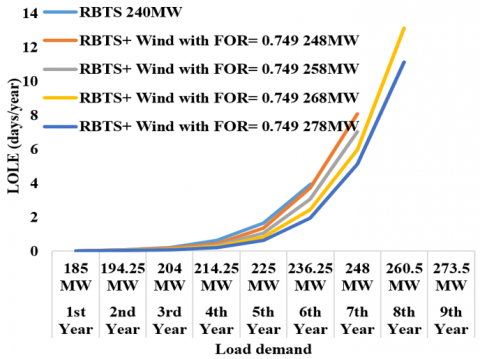Figure 6. Variation of LOLE and load demand for RBTS incorporated Wind system using standard load forecasting

## 7.png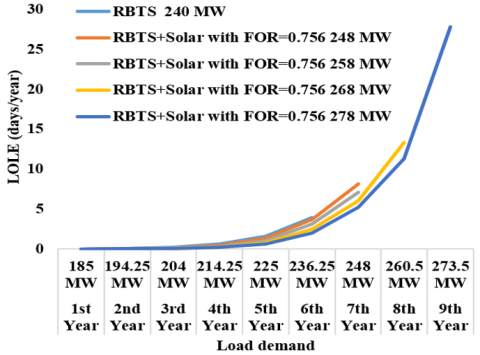Figure 7. Variation of LOLE and load demand for RBTS incorporated Solar system using standard load forecasting

## 8.png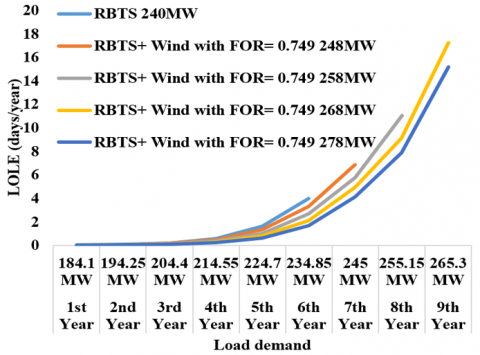Figure 8. Variation of LOLE and load demand for RBTS incorporated Wind system using Time series load forecasting

## 9.png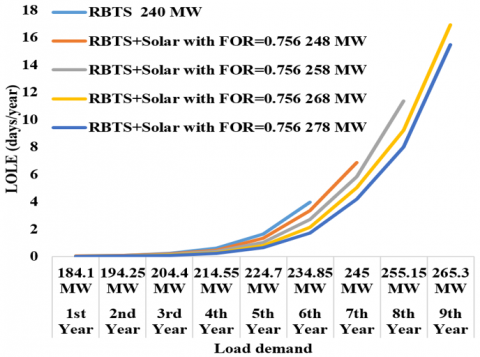Figure 9. Variation of LOLE and load demand for RBTS incorporated Solar system using Time series load forecasting

7.2 Cost optimization results with sensitivity analysis for solar and wind system

The generation system considered is Solar and Wind system. The capacity of Solar PV system is 2494 kW and the capacity of Wind is 546 kW. The total cost i.e. net present cost was analyzed based on the system components used. The Solar and Wind system are operated with converter and battery components. To analyze the system, the residential load was considered as 1971.19 kWh/day with peak load of 139.94 kW. This residential load data is taken from HOMER software to understand the levelized cost and net present cost of the system. The capital cost, replacement cost, operating cost and salvage for Generic 3 kW Wind and Flat plate Solar PV with battery and converter was shown in Table 10. This table describes the net present cost for the system. The levelized cost of Solar and Wind are 0.00229 \$/kWh and 0.0294 \$/kWh.

The multiplier for every component was set to 0.75 and 1. Table 11 shows when the multiplier set to 1. The total Net present cost is \$20,72,954. If the multiplier set to 0.75, then the capital and operating cost reduced to 25%. During this case, there was variation in the net present cost and cost of energy. The Net present cost for this proposed optimization model was reduced from \$ 27,60,000 to \$20,72,954. The best solution from several iteration in HOMER software is highlighted in Table 11. The below result shows the best solution of net present cost and levelized cost of energy calculated from various iteration using HOMER software. Similarly, Levelized cost of Energy reduced from \$ 0.296 to \$0.223 when multiplier set to 1 and when multiplier set to 0.75 then the levelized cost of Energy \$ 0.167 and the net present cost is \$15,50,000. The Table 11 shows the Sensitivity analysis with variation in Capital and operating cost of the system. Table 11. Sensitivity analysis with variation in capital and operating cost  System parameters Multiplier=1 Multiplier=1 Multiplier=0.75 Base model Proposed model Proposed model Net Present cost \$ 27,60,000 \$20,72,954 \$ 15,50,000 Levelized cost of Energy (for kWh) \$0.296 \$ 0.223 \$0.167 8. Conclusion The reliability and cost are evaluated for the system consists of Solar and Wind. The adequacy is assessed in the system which is analyzed using standard load forecasting and time series load forecasting techniques. There were better reliability and cost of the system in the result of the methodology utilized. In the proposed methodology of adequacy assessment, the reliability indices like Loss of Load Expectation are evaluated. The results show that the RBTS generation system will satisfy the load demand only for 6 years. The solar/wind capacities of 38 MW are embedded in the RBTS system to meet the load demand in the 9th year. So, in this 9th year, the LOLE for wind and solar using standard load forecasting technique is 27.2427 days/year and 27.75952 and using time series load forecasting technique is 15.18512 days/year and 15.44692 days/year. The maximum negative and positive errors during measurement using the time series forecasting method are -0.486486% and 0.196078%. The latest load forecast is evaluated using this method based on the observed load demand. The methodology developed will helps to analyze the reliability of the system for various cases. The cost optimization for Solar and Wind were conducted using HOMER software. The result shows that the Levelized cost is minimized from$ 0.296 to \$ 0.223. The main innovation for conducting this research is the reliability evaluated using forecasting technique which can be used for generation planning and cost optimization gives the best solution with less cost for the system.

Acknowledgements

My sincere thanks to my supervisor, Dr. P.K. Dhal, for his suggestions given for my research work. It is worth mentioning that his guidelines and conceptual ideas at every stage have shaped my work in the right direction.

References

 Gami, D., Sioshansi, R., Denholm, P. (2017). Data challenges in estimating the capacity value of solar photovoltaics. IEEE Journal of Photovoltaics, 7(4): 1065-1073. https://doi.org/10.1109/JPHOTOV.2017.2695328

 Kumar, S., Saket, R. K., Dheer, D.K., Holm-Nielsen, J. B., Sanjeevikumar, P. (2020). Reliability enhancement of electrical power system including impacts of renewable energy sources: a comprehensive review. IET Generation, Transmission & Distribution, 14(10): 1799-1815. https://doi.org/10.1049/iet-gtd.2019.1402

 Adefarati, T., Bansal, R.C., Justo, J.J. (2017). Reliability and economic evaluation of a microgrid power system. Energy Procedia, 142: 43-48. https://doi.org/10.1016/j.egypro.2017.12.008

 Adefarati, T., Bansal, R.C. (2017). Reliability and economic assessment of a microgrid power system with the integration of renewable energy resources. Applied Energy, 206: 911-933. https://doi.org/10.1016/j.apenergy.2017.08.228

 Adefarati, T., Bansal, R.C. (2019). Reliability, economic and environmental analysis of a microgrid system in the presence of renewable energy resources. Applied Energy, 236: 1089-1114. https://doi.org/10.1016/j.apenergy.2018.12.050

 Adefarati, T., Bansal, R.C. (2017). Reliability assessment of distribution system with the integration of renewable distributed generation. Applied Energy, 185: 158-171. https://doi.org/10.1016/j.apenergy.2016.10.087

 Hahn, B., Durstewitz, M., Rohrig, K. (2007). Reliability of wind turbines-Experience of 15 years with 1,500 WTs, wind energy. In Proc. Euromech Colloquium, Springer, Berlin (Vol. 101).

 Peyghami, S., Palensky, P., Blaabjerg, F. (2020). An overview on the reliability of modern power electronic based power systems. IEEE Open Journal of Power Electronics, 1: 34-50. https://doi.org/10.1109/OJPEL.2020.2973926

 Douglas, A.P., Breipohl, A.M., Lee, F.N., Adapa, R. (1998). Risk due to load forecast uncertainty in short term power system planning. IEEE Transactions on Power Systems, 13(4): 1493-1499. https://doi.org/10.1109/59.736296

 Billinton, R., Huang, D. (2008). Effects of load forecast uncertainty on bulk electric system reliability evaluation. IEEE Transactions on Power Systems, 23(2): 418-425. https://doi.org/10.1109/TPWRS.2008.920078

 El-Sheikhi, F.A., Billinton, R. (1991). Load forecast uncertainty consideration in generating unit preventive maintenance scheduling for single systems. 1991 Third International Conference on Probabilistic Methods Applied to Electric Power Systems, London, UK, pp. 241-245.

 Singh, A.K., Khatoon, S., Muazzam, M., Chaturvedi, D.K. (2012). Load forecasting techniques and methodologies: A review. In 2012 2nd International Conference on Power, Control and Embedded Systems, Allahabad, India, pp. 1-10. https://doi.org/10.1109/ICPCES.2012.6508132

 Allahnoori, M., Kazemi, S., Abdi, H., Keyhani, R. (2014). Reliability assessment of distribution systems in presence of microgrids considering uncertainty in generation and load demand. Journal of Operation and Automation in Power Engineering, 2(2): 113-120.

 Karki, R., Hu, P. Billinton, R. (2010). Reliability evaluation considering wind and hydro power coordination. IEEE Trans. Power Systems, 25: 685-693. https://doi.org/10.1109/TPWRS.2009.2032758

 Hu, P., Karki, R., Billinton, R. (2009). Reliability evaluation of generating systems containing wind power and energy storage. IET Generation, Transmission & Distribution, 3(8): 783-791. https://doi.org/10.1049/iet-gtd.2008.0639

 Ehnberg, S.J., Bollen, M.H. (2005). Reliability of a small power system using solar power and hydro. Electric Power Systems Research, 74(1): 119-127. https://doi.org/10.1016/j.epsr.2004.09.009

 Arabali, A., Ghofrani, M., Etezadi-Amoli, M., Fadali, M.S. (2014). Stochastic performance assessment and sizing for a hybrid power system of solar. Wind/Energy Storage. IEEE Transactions on Sustainable Energy, 5(2): 363-371. https://doi.org/10.1109/TSTE.2013.2288083

 Chowdhury, A.A. (2005). Reliability models for large wind farms in generation system planning. IEEE Power Engineering Society General Meeting, San Francisco, CA, USA, pp. 1926-1933. https://doi.org/10.1109/PES.2005.1489161

 Cheng, L., Liu, M., Sun, Y., Ding, Y. (2013). A multi-state model for wind farms considering operational outage probability. Journal of Modern Power Systems and Clean Energy, 1(2): 177-185. https://doi.org/10.1007/s40565-013- 0025-z

 Fathima, H., Palanisamy, K. (2015). Optimized sizing, selection, and economic analysis of battery energy storage for grid-connected wind-PV hybrid system. Modelling and Simulation in Engineering. https://doi.org/10.1155/2015/713530

 Akram, U., Khalid, M., Shafiq, S. (2017). An innovative hybrid wind-solar and battery-supercapacitor microgrid system-Development and optimization. IEEE Access, 5: 25897-25912. https://doi.org/10.1109/ACCESS.2017.2767618

 Hosseinalizadeh, R., Shakouri, H., Amalnick, M.S., Taghipour, P. (2016). Economic sizing of a hybrid (PV–WT–FC) renewable energy system (HRES) for stand-alone usages by an optimization-simulation model: Case study of Iran. Renewable and Sustainable Energy Reviews, 54: 139-150. https://doi.org/10.14288/1.0306939

 Arabali, A. Ghofrani, M., Etezadi-Amoli, M., Fadali, M.S. (2014). Stochastic performance assessment and sizing for a hybrid power system of solar/wind/energy storage. IEEE Trans. Sustain. Energy, 5(2): 363-371. https://doi.org/10.1109/TSTE.2013.2288083

 Bett, P.E., Thornton, H.E. (2016). The climatological relationships between wind and solar energy supply in Britain. Renewable Energy, 87: 96-110. https://doi.org/10.1016/j.renene.2015.10.006

 Renugadevi, V. (2017). An approach to solar power tree. In 2017 IEEE International Conference on Electrical, Instrumentation and Communication Engineering (ICEICE), Karur, India, pp. 1-3. https://doi.org/10.1109/ICEICE.2017.8191921

 Chaurasiya, P.K., Warudkar, V., Ahmed, S. (2019). Wind energy development and policy in India: A review. Energy Strategy Reviews, 24: 342-357. https://doi.org/10.1016/j.esr.2019.04.010

 Vijayalaxmi, D., Karjagi, Β. (2015). Modeling and analysis of RBTS IEEE-6 BUS system based on Markov Chain. Int J Eng Res Gen Sci, 3(2): 686-696.

 Simulation of Solar radiation and wind speed data. https://www.renewables.ninja/.

 Ali Kadhem, A., Abdul Wahab, N.I., Abdalla, A.N. (2019). Wind energy generation assessment at specific sites in a Peninsula in Malaysia based on reliability indices. Processes, 7(7): 399. https://doi.org/10.3390/pr7070399

 Sulaeman, S., Benidris, M., Mitra, J. (2015). Modeling the output power of pv farms for power system adequacy assessment. In 2015 North American Power Symposium (NAPS), Charlotte, NC, USA, pp. 1-6. https://doi.org/10.1109/NAPS.2015.7335216

 Lalitha, M.P., Reddy, P.H., Naidu, P.J. (2014). Generation reliability evaluation of wind energy penetrated power system. 2014 International Conference on High Performance Computing and Applications (ICHPCA), Bhubaneswar, India, pp. 1-4. https://doi.org/10.1109/ICHPCA.2014.7045371

 Prasad, C.S., Raikar, S., Prakash, M. (2014). To study the adequacy assessment of generation system. International Journal of Advanced Research in Electrical, Electronics and Instrumentation Engineering, 3(1): 6869-6880.

 Roy, A., Sahoo, S.S., Chatterjee, K. (2017). A reliability assessment model of a wind farm for generation adequacy studies of wind integrated power system. In 2017 Third International Conference on Science Technology Engineering & Management (ICONSTEM), Chennai, India, pp. 566-570. https://doi.org/10.1109/ICONSTEM.2017.8261386

 Jiang, W., Yan, Z., Feng, D. (2009). A review on reliability assessment for wind power. Renewable and Sustainable Energy Reviews, 13(9): 2485-2494. https://doi.org/10.1016/j.rser.2009.06.006

 Bansal, M., Khatod, D.K., Saini, R.P. (2014). Modeling and optimization of integrated renewable energy system for a rural site. 2014 International Conference on Reliability Optimization and Information Technology (ICROIT), Faridabad, India, pp. 25-28. https://doi.org/10.1109/ICROIT.2014.6798289

 Babaei, A., Gholizadeh, B., Khaliliaqdam, N., Gilanipour, J. (2013). Optimizing and economical assessment of the utilization of photovoltaic systems in residential buildings: the case of Sari station, northern Iran. International Journal of Agriculture, 3(1): 65-71.

 Ajao, K.R., Oladosu, O.A., Popoola, O.T. (2011). Using HOMER power optimization software for cost benefit analysis of hybrid -solar power generation relative to utility cost in Nigeria. International Journal of Research and Reviews in Applied Sciences, 7(1): 96-102.

 Tudu, B., Mandal, K.K., Chakraborty, N. (2014). Optimal design and performance evaluation of a grid independent hybrid micro hydro-solar-wind-fuel cell energy system using meta-heuristic techniques. 2014 1st International Conference on Non-Conventional Energy (ICONCE 2014), Kalyani, India, pp. 89-93. https://doi.org/10.1109/ICONCE.2014.6808696